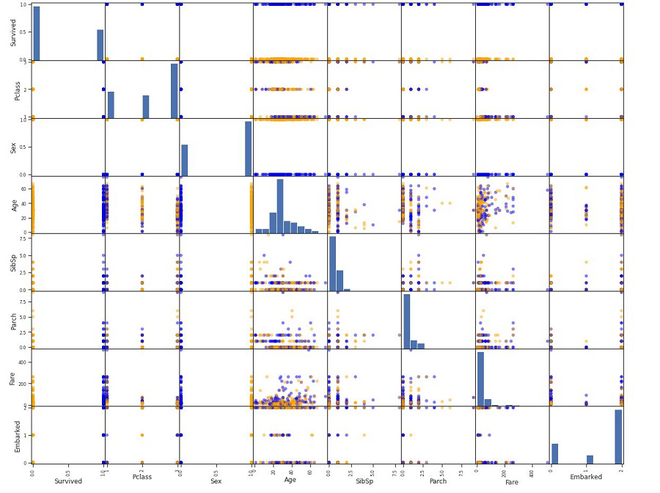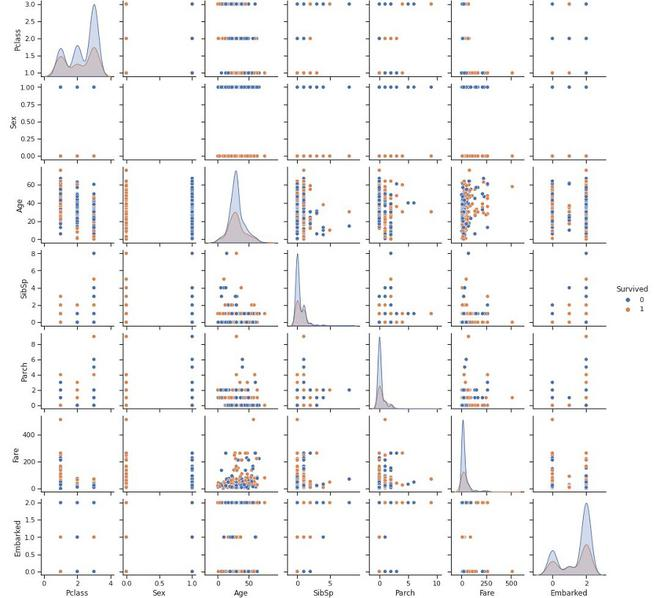# Scatter Plot Matrix

• Last Updated : 21 Nov, 2021

In a dataset, for k set of variables/columns (X1, X2, ….Xk), the scatter plot matrix plot all the pairwise scatter between different variables in the form of a matrix.

Scatter plot matrix answer the following questions:

• Are there any pair-wise relationships between different variables? And if there are relationships, what is the nature of these relationships?
• Are there any outliers in the dataset?
• Is there any clustering by groups present in the dataset on the basis of a particular variable?

For k variables in the dataset, the scatter plot matrix contains k rows and k columns. Each row and column represents as a single scatter plot. Each individual plot (i, j) can be defined as:

• Vertical Axis: Variable Xj
• Horizontal Axis: Variable Xi

Below are some important factors we consider when plotting the Scatter plot matrix:

• The plot lies on the diagonal is just a 45 line because we are plotting here Xi vs Xi. However, we can plot the histogram for the Xi in the diagonals or just leave it blank.
• Since Xi vs Xj is equivalent to Xj vs Xi with the axes reversed, we can also omit the plots below the diagonal.
• It can be more helpful if we overlay some line plot on the scattered points in the plots to give more understanding of the plot.
• The idea of the pair-wise plot can also be extended to different other plots such as qunatile-quantile plots or bihistogram.

### Implementation

• For this implementation, we will be using the Titanic dataset. This dataset can be downloaded from Kaggle. Before plotting the scatter matrix, we will be performing some preprocessing operations on the dataframe to obtain it into the desired form.

## Python3

 `import` `pandas as pd` `import` `numpy as np` `import` `seaborn as sns` `import` `matplotlib.pyplot as plt` `%` `matplotlib inline`   `# load titanic dataset` `titanic_dataset ``=` `pd.read_csv(``'tested.csv.xls'``)` `titanic_dataset.head()` `# Drop some unimportant columns in the dataset.` `titanic_dataset.drop([``'Name'``, ``'Ticket'``,``'Cabin'``,``'PassengerId'``],axis``=``1``, inplace``=``True``)`   `# check for different data types` `titanic_dataset.dtypes`   `# print unique values of dataset` `titanic_dataset[``'Embarked'``].unique()` `titanic_dataset[``'Sex'``].unique()`   `# Replace NAs with mean` `titanic_dataset.fillna(titanic_dataset.mean(), inplace``=``True``)`   `# convert some column into integer for representation in ` `# scatter matrix` `titanic_dataset[``"Sex"``] ``=` `titanic_dataset[``"Sex"``].cat.codes` `titanic_dataset[``"Embarked"``] ``=` `titanic_dataset[``"Embarked"``].cat.codes`   `titanic_dataset.head()`   `# plot scatter matrix using pandas and matplotlib` `survive_colors ``=` `{``0``:``'orange'``, ``1``:``'blue'``}` `pd.plotting.scatter_matrix(titanic_dataset,figsize``=``(``20``,``20``),grid``=``True``,` `                           ``marker``=``'o'``, c``=` `titanic_dataset[``'Survived'``].``map``(colors))`     `# plot scatter matrix using seaborn` `sns.set_theme(style``=``"ticks"``)` `sns.pairplot(titanic_dataset, hue``=``'Survived'``)`

```PassengerId    Survived    Pclass    Name    Sex    Age    SibSp    Parch    Ticket    Fare    Cabin    Embarked
0    892    0    3    Kelly, Mr. James    male    34.5    0    0    330911    7.8292    NaN    Q
1    893    1    3    Wilkes, Mrs. James (Ellen Needs)    female    47.0    1    0    363272    7.0000    NaN    S
2    894    0    2    Myles, Mr. Thomas Francis    male    62.0    0    0    240276    9.6875    NaN    Q
3    895    0    3    Wirz, Mr. Albert    male    27.0    0    0    315154    8.6625    NaN    S
4    896    1    3    Hirvonen, Mrs. Alexander (Helga E Lindqvist)    female    22.0    1    1    3101298    12.2875    NaN    S```
```PassengerId      int64
Survived         int64
Pclass           int64
Sex             object
Age            float64
SibSp            int64
Parch            int64
Fare           float64
Embarked        object
dtype: object```
```Survived    Pclass    Sex    Age    SibSp    Parch    Fare    Embarked
0    0    3    1    34.5    0    0    7.8292    1
1    1    3    0    47.0    1    0    7.0000    2
2    0    2    1    62.0    0    0    9.6875    1
3    0    3    1    27.0    0    0    8.6625    2
4    1    3    0    22.0    1    1    12.2875    2```Matplotlib Scatter matrixSeaborn Scatter matrix

### References:

My Personal Notes arrow_drop_up
Recommended Articles
Page :